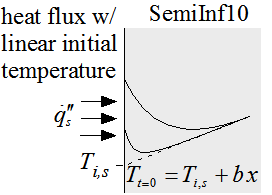Contents - Index

Linear initial temperature and constant heat fluxFunction SemiInf10(T_i_s, b, alpha, k, q''_dot_s, x, time) returns the temperature within a semi-infinite body with a linear initial temperature exposed to a constant heat flux.

The calling protocol is:

T = SemiInf10(T_i_s, b, alpha, k, q``_dot_s, x, t)

Inputs:
T_i_s = initial surface temperature of the material [C] or [K]
b = temperature gradient [C/m] or [K/m]
alpha = thermal diffusivity [m^2/s]
k = thermal conductivity [W/m-K]
q``_dot_s = surface heat flux [W/m^2]
x = position relative to surface [m]
time = time relative to beginning of surface disturbance [s]

This function can be used with English units set in EES.  In this case, T_i_s are in [F] or [R], b is in [F/ft] or [R/ft], alpha is in [ft^2/hr], k is in [Btu/hr-ft-R], q``_dot_s is in [Btu/hr-ft^2], x is in [ft], and time is in [s].

Outputs:
T is the temperature in [C] or [K] (or [F] or [R] in English units)

Zhuang JR, Werner K, Schlünder E-U (1995) Study of the analytical solution to the heat transfer problem and surface temperature in semi-infinite body with a constant flux at the surface and an initial temperature distribution. Heat Mass Transfer 30(3):183-186.

Example:
\$UnitSystem SI Mass J K Pa Radian
T_s_i=100 [K]
b=10 [K/m]
alpha=1e-3 [m^2/s]
k=100 [W/m-K]
q``_dot_s=100000 [W/m^2]
x=0.1 [m]
t=10 [s]
Temp=semiinf10(T_s_i,b,alpha,k,q``_dot_s,x,t)
{Solution:
T=141.3 [K]}

Transient Conduction Index